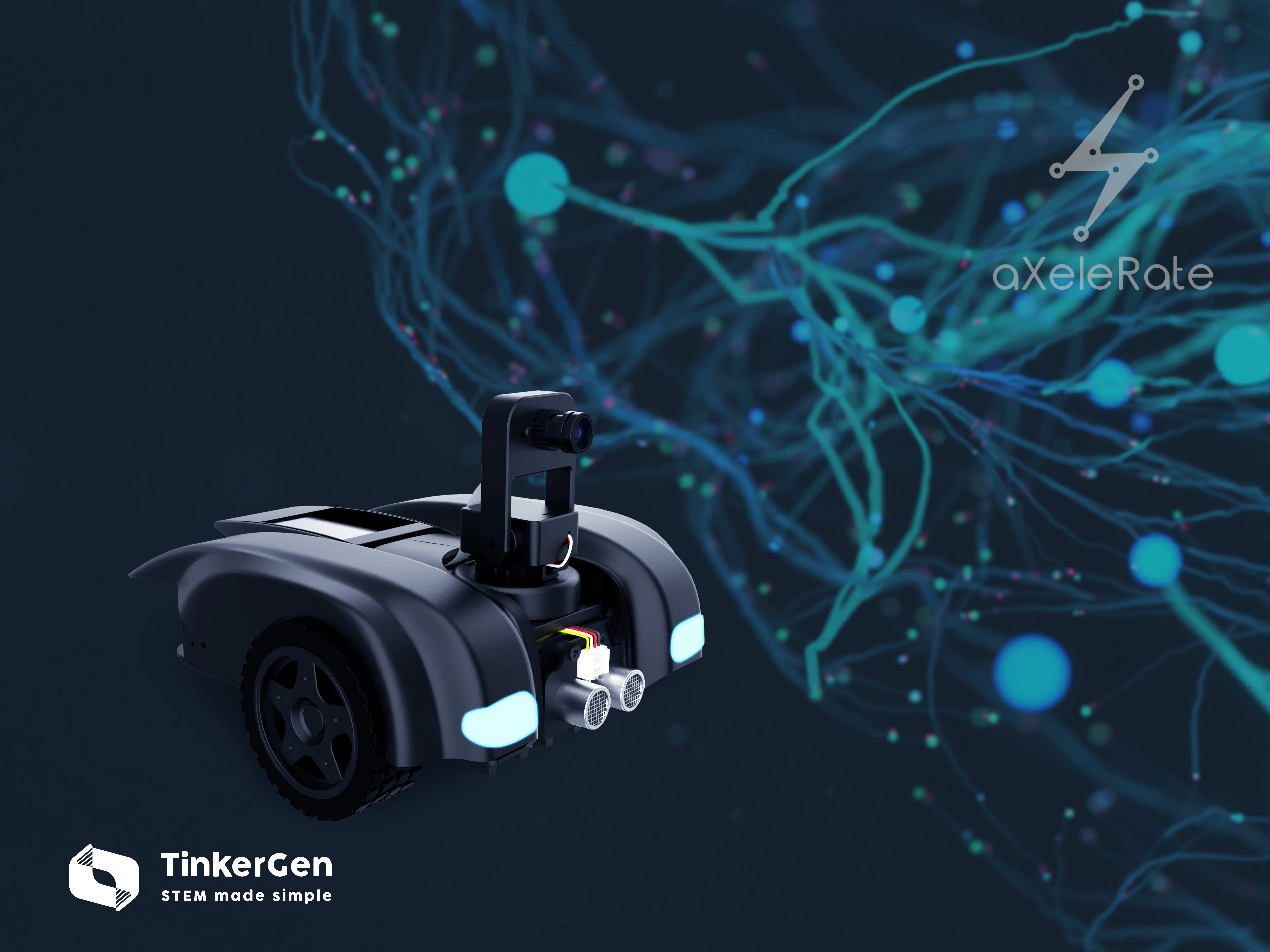# aXeleRate - Keras-Based Framework for AI on the Edge

Train your machine learning models in Google Colab and easily optimize them for hardware accelerated inference!

BeginnerProtip2 hours997## Things used in this project

### Hardware components

 Seeed M.A.R.K kit
×1

### Software apps and online services

 aXeleRate

## Code

### Micropython person following example

MicroPython
```import sensor,image,lcd, os, time, utime
from maix_motor import Maix_motor
import KPU as kpu

def find_center():
img = sensor.snapshot()
img = img.resize(224,224)
img= img.rotation_corr(z_rotation=90.0)
a = img.pix_to_ai()
if code:
for i in code:
a=img.draw_rectangle(i.rect(),color = (0, 255, 0))
a = img.draw_string(i.x(),i.y(), classes[i.classid()], color=(255,0,0), scale=3)
x_center = i.x()+i.w()/2
print(x_center)
a = lcd.display(img)
return x_center
else:
a = lcd.display(img)
return -1

def scan_pan():
Maix_motor.motor_run(0, 0, 0)
Maix_motor.servo_angle(2, 110)
for i in range(0, 180, 5):
pos = find_center()
if pos >= 100 and pos <= 124:
Maix_motor.servo_angle(1, 90)
if i >= 90:
Maix_motor.motor_right(40, 0)
Maix_motor.motor_left(-40, 0)
utime.sleep_ms(((i - 90) * 12))
if i < 90:
Maix_motor.motor_right(-40, 0)
Maix_motor.motor_left(40, 0)
utime.sleep_ms(((90 - i) * 12))
Maix_motor.motor_motion(1, 1, 0)
time.sleep(0.3)
return True
else:
Maix_motor.servo_angle(1, i)

def scan_tilt():
#Maix_motor.motor_run(0, 0, 0)
Maix_motor.servo_angle(1, 90)
for i in range(100, 140, 2):
pos = find_center()
if pos > 0:
return True
else:
Maix_motor.servo_angle(2, i)

def scan_rotate():
Maix_motor.servo_angle(1, 90)
Maix_motor.servo_angle(2, 110)
Maix_motor.motor_motion(1, 3, 0)
while True:
pos = find_center()
if pos > 0:
break

lcd.init()
sensor.reset()
sensor.set_pixformat(sensor.RGB565)
sensor.set_framesize(sensor.QVGA)
sensor.set_vflip(1)
sensor.run(1)
Maix_motor.servo_angle(1, 90)
Maix_motor.servo_angle(2, 110)
DEBUG = 0
classes = ["person"]
anchor = (0.57273, 0.677385, 1.87446, 2.06253, 3.33843, 5.47434, 7.88282, 3.52778, 9.77052, 9.16828)
a = kpu.init_yolo2(task, 0.3, 0.3, 5, anchor)
while(True):
x_center = find_center()
if not DEBUG:
if x_center >= 100 and x_center <= 124: Maix_motor.motor_motion(1, 1, 0)
if x_center < 100 and x_center > 0: Maix_motor.motor_motion(1, 4, 0)
if x_center > 124: Maix_motor.motor_motion(1, 3, 0)
if x_center < 0:
if not scan_tilt():
scan_pan()
if not scan_pan():
scan_rotate()